• Uniform capital allowance system – Changing a depreciating asset's effective life

From 1 July 2001, uniform capital allowance (UCA) rules will apply to most depreciating assets. Taxpayers generally calculate deductions for the decline in value of their depreciating assets using these rules.

Under UCA rules, deductions for the decline in value of depreciating assets are generally calculated on the basis of effective life. You can choose to recalculate the effective life of an asset in certain circumstances where the effective life you have been using is no longer accurate. If you make an improvement to an asset, you may be obliged to recalculate its effective life.

Simplified depreciation rules

If you are using the simplified depreciation rules, you generally you won't use UCA rules. Under the simplified depreciation rules, you can claim an immediate deduction for most depreciating assets costing less than \$30,000. Business with a turnover of up to \$10 million can also claim a deduction for each asset purchased and first used or installed ready for use, up to the following thresholds:

• \$30,000, from 7:30 pm (AEDT) on 2 April 2019 until 30 June 2020
• \$25,000, from 29 January 2019 until before 7:30 pm (AEDT) on 2 April 2019
• \$20,000, before 29 January 2019.

Your business clients can't immediately claim a deduction for individual assets that cost \$30,000 or more. They can continue to deduct these over time using the small business pool or general depreciation rules, depending on their turnover.

You can use the simplified depreciation rules if you are a small business entity (2007–08 and later years).

You must use the simplified depreciation rules for income years where you were in the simplified tax system (2006–07 and earlier years).

For more details refer to Simplified depreciation – rules and calculations

Recalculating effective life

For plant acquired from 21 September 1999 and for depreciating assets acquired from 1 July 2001, the calculation of decline in value is generally based on the effective life of the plant or asset rather than on the accelerated rates of depreciation that were previously available.

You can choose to recalculate the effective life of a depreciating asset if the nature of your use of that asset changes and those changed circumstances make your current estimate inaccurate. Your choice to recalculate applies where:

• you acquired the depreciating asset, or its construction began from 1 July 2001
• you acquired the plant, or its construction began between 21 September 1999 and 30 June 2001.

You can reassess effective life, regardless of whether you made the existing estimate yourself, or adopted the effective life specified by the Commissioner of Taxation

You can only make a new estimate of effective life after the end of the income year in which you first started to use the asset for any purpose (including a non-income producing purpose).

When you must recalculate effective life

You must recalculate the effective life of a depreciating asset if the asset's cost increases by at least 10% in an income year and you:

• self-assessed the effective life of the asset
• used the Commissioner's determination of effective life and the prime cost method to calculate the asset's decline in value.

You may conclude that the effective life has not changed.

There are special rules for recalculating effective life where depreciating assets are transferred between associates.

When you can't recalculate effective life

You can't recalculate the effective life of a depreciating asset where:

• accelerated depreciation rates are available for the asset
• the asset is an intangible asset, such as a licence, copyright or software
• you simply miscalculated the existing effective life – so your circumstances of use have not changed. In this case, you may be able to correct the miscalculation by seeking an amendment of your existing estimate from the time allowed under the amendment provisions of the law.

Changed circumstances

Changed circumstances that could result in your estimate of effective life becoming inaccurate include:

• your use of the asset turns out to be more or less rigorous than expected
• the asset is scrapped because of a downturn in demand for the goods or services it is used to produce
• legislation prevents the asset's continued use
• new technology makes the asset redundant.

Example – changed circumstances

A depreciating asset used to produce insecticide has a remaining effective life of 10 years. The government decides that use of the insecticide is to be phased out and legislates to end production within two years.

The depreciating asset can't be used for any other purpose so will be scrapped in two years. The effective life of the depreciating asset may then be re-estimated to reflect the loss of eight years of its previously estimated effective life.

End of example

How to make a new estimate of effective life

The process of recalculating effective life is the same as the process of estimating effective life. To make a new estimate, you estimate the period (in years) the asset can be used for income-producing purposes by any entity from the time you first started to use the asset for any purpose. The period is based on your expected use of the asset and assumes that it is maintained in reasonably good order and condition.

The new estimate of effective life takes effect for the year in which you make it.

Example – making a new estimate of effective life

DA Pty Ltd acquires a depreciating asset on 1 July 2001 and starts to use it on the same day. The company works out that the effective life of the asset is seven years. During 2001–02, the company is advised that use of the asset will be prohibited by law from 1 July 2003.

The effective life can't be recalculated for 2001–02 because this is the year in which the company started to use the asset. However, for 2002–03 the company may recalculate the effective life at two years. The recalculated effective life is worked out from the time the company first started to use the asset to the date when it can no longer be used for income-producing purposes.

End of example

Calculating a depreciating asset's decline in value after its effective life has been recalculated

If you are using the diminishing value method to calculate the decline in value of the asset, the new estimate of effective life is used in the diminishing value formula: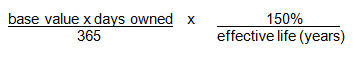if you start to hold the asset prior to 10 May 2006, or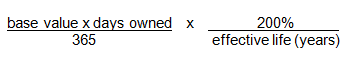if you start to hold the asset on or after 10 May 2006.

Under the prime cost method, an adjusted prime cost formula must be used from the year in which the effective life is recalculated: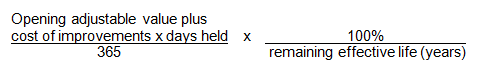In the adjusted formula, the asset's opening adjustable value (plus the cost of any improvements made to the asset during the year) is substituted for the asset's cost. For effective life, you substitute the period of the recalculated effective life that remains at the start of the year in which you make the new estimate.

Example – diminishing value method

Using the facts of the previous example, assume that DA Pty Ltd chose to use the diminishing value method to calculate the decline in value of the asset and the cost of the asset is \$10,000. The company's deduction for 2001–02 would be \$2,143 calculated as follows: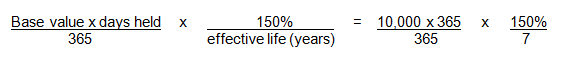For 2002–03, the company recalculates the effective life for the asset at two years. The asset's opening adjustable value for 2002–03 is \$7,857 (that is, \$10,000 less \$2,143). DA Pty Ltd can claim depreciation of \$5,893 for the year, based on the new estimate of effective life of two years as follows: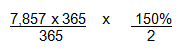Example (prime cost method)

Assume that DA Pty Ltd uses the prime cost method to calculate the decline in value of the asset. For 2001–02, the deduction for the decline in value of the asset would be \$1,429 calculated as follows: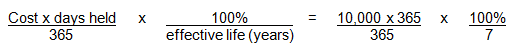For 2002–03 the company recalculates the effective life for the asset at two years.

Under the prime cost method, an adjusted formula must be used to calculate the decline in value for this and later years. The asset's remaining effective life, worked out as at the start of the income year, is one year. This figure is used instead of the earlier estimate of the asset's effective life and the opening adjustable value of \$8,571 (that is, \$10,000 less \$1,429) is substituted for the asset's cost. The deduction for 2002–03 would be \$8,571 calculated as follows: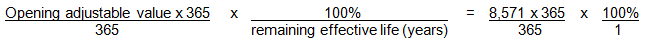End of example